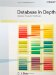# More on Relational Assignment

 The assignment operator ":=" resembles the equality comparison operator "=" in that it applies to every type, and relation types are no exception. Relational assignment in particular resembles "=" and the other comparison operators from the previous section in another respect as well: it isn't part of the relational algebra. Why not? Because its target must be, very specifically, a relvar, not a relation (relvars aren't part of the relational algebra either there's no notion of updating in the relational algebra as such, and "variable" means "updatable"). Nevertheless, I want to say a little more about relational assignment in this chapter; to be specific, I want to examine the INSERT, DELETE, and UPDATE shorthands a little more closely. As you know, these operators are just shorthand for certain relational assignments but now I'm in a position to explain just what the "longhand" versions look like, in terms of appropriate algebraic operators. I'll do this by showing some simple examples. First, relational assignment in general looks like this: ` R := rx ` R here is a relvar and rx is a relational expression of the same type as R. The effect is to assign the relation r that's denoted by the expression rx to the relvar R (and I remind you from the exercises in Chapter 2 that after the assignment, the boolean expression R = r is required to evaluate to TRUE: The Assignment Principle). Here's a simple example: ` S := RELATION { TUPLE { SNO SNO('S1'), SNAME NAME('Smith'), STATUS 20, CITY 'London' } , TUPLE { SNO SNO('S2'), SNAME NAME('Jones'), STATUS 10, CITY 'Paris' } , TUPLE { SNO SNO('S3'), SNAME NAME('Blake'), STATUS 30, CITY 'Paris' } , TUPLE { SNO SNO('S4'), SNAME NAME('Clark'), STATUS 20, CITY 'London' } , TUPLE { SNO SNO('S5'), SNAME NAME('Adams'), STATUS 30, CITY 'Athens' } } ; ` (As we saw in Chapter 3, the expression on the right here is another relation selector invocation; in fact, it's a relation literal.) Now I can turn to INSERT, DELETE, and UPDATE. Let relvar PQ be defined as follows: ` VAR PQ BASE RELATION { PNO PNO, QTY QTY } KEY { PNO } ; ` Here's a possible INSERT on this relvar: ` INSERT PQ ( SUMMARIZE SP PER ( P { PNO } ) ADD ( SUM ( QTY ) AS QTY ) ) ; ` And here's the "longhand" assignment equivalent: ` PQ := PQ UNION ( SUMMARIZE SP PER ( P { PNO } ) ADD ( SUM ( QTY ) AS QTY ) ) ; ` In other words, the INSERT works by forming the union, pq say, of the old value of relvar PQ and the relation denoted by the SUMMARIZE invocation, and then assigning that relation pq to relvar PQ. (By the way, I'm assuming here that it's not an error to insert a tuple that already exists in the target. If it is, that UNION in the expansion should be replaced by D_UNION.) Next, a DELETE example: ` DELETE S WHERE CITY = 'Athens' ; ` Longhand equivalent: ` S := S WHERE NOT ( CITY = 'Athens' ) ; ` Finally, an UPDATE example: ` UPDATE P WHERE CITY = 'London' ( WEIGHT := 2 * WEIGHT , CITY := 'Oslo' ) ; ` Longhand equivalent: ` P := WITH ( P WHERE CITY = 'London' ) AS R1 , ( EXTEND R1 ADD ( 2 * WEIGHT AS NEW_WEIGHT, 'Oslo' AS NEW_CITY ) ) AS R2 , R2 { ALL BUT WEIGHT, CITY } AS R3 : R3 RENAME ( NEW_WEIGHT AS WEIGHT, NEW_CITY AS CITY ) ; ` This one needs a little more explanation. First, R1 is the set of tuples to be updated (loosely speaking see the section Chapter 4). Next, we extend each tuple in R1 with the applicable new WEIGHT and CITY values; that's R2. Then we throw away the old WEIGHT and CITY values (that's R3). Finally, we rename NEW_WEIGHT and NEW_CITY as WEIGHT and CITY, respectively, and assign the resulting relation to relvar P. Notice the use of the "multiple" forms of EXTEND and RENAME in this example.Database in Depth: Relational Theory for Practitioners
ISBN: 0596100124
EAN: 2147483647
Year: 2006
Pages: 127
Authors: C.J. Date

Similar book on Amazon